# Consider the following function fx) = 2x arctan (a) Find the critical numbers off. (Enter your...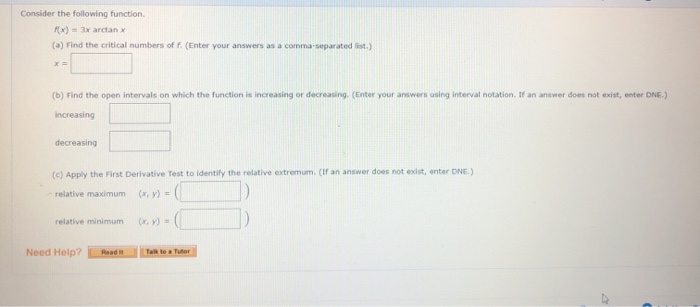Consider the following function fx) = 2x arctan (a) Find the critical numbers off. (Enter your answers as a comma-separated list.) (6) Find the open intervals on which the function is increasing or decreasing (Enter your answers using interval notation. If an answer does not exist, enter DNE.) increasing decreasing (c) Apply the First Derivative Test to identify the relative extremum. (If an answer does not exist, enter ONE.) - relative maximum ( ) = relative minimum (X,Y)=( Need Help? Read it all to a Tutor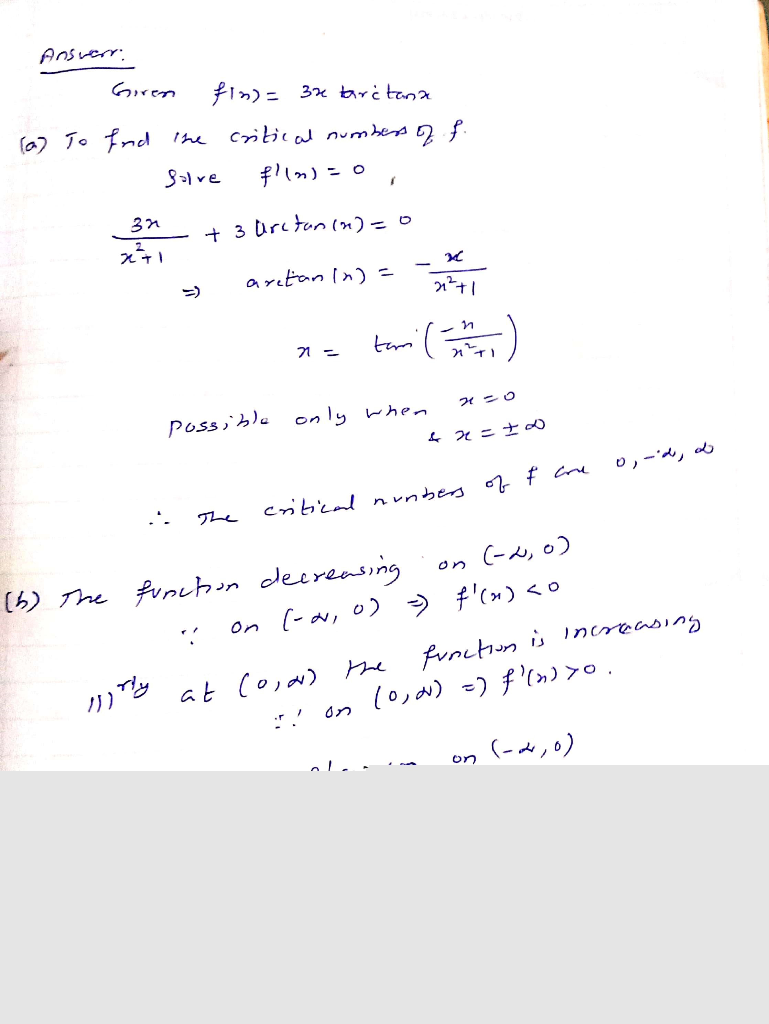#### Earn Coin

Coins can be redeemed for fabulous gifts.

Similar Homework Help Questions
• ### Consider the following function. f(x) = 5x + 81 - 2 (a) Find the critical numbers...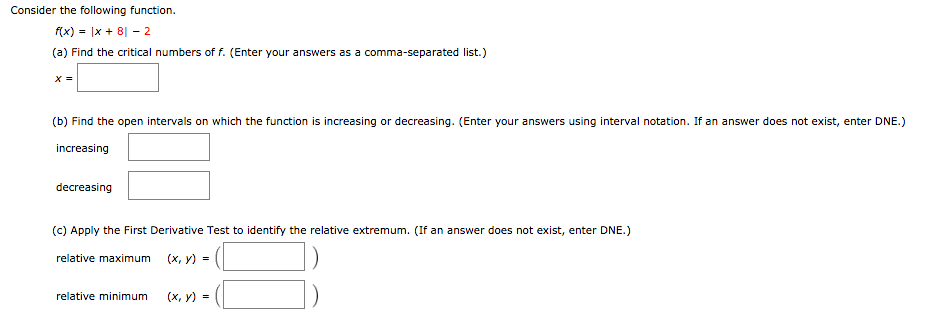Consider the following function. f(x) = 5x + 81 - 2 (a) Find the critical numbers of f. (Enter your answers as a comma-separated list.) (b) Find the open intervals on which the function is increasing or decreasing. (Enter your answers using interval notation. If an answer does not exist, enter DNE.) increasing decreasing (c) Apply the First Derivative Test to identify the relative extremum. (If an answer does not exist, enter DNE.) relative maximum (x,y) = relative minimum (X,Y)...

• ### -15 points LARCALC11 3.3.019. Consider the following function. f(x) = x2 - 10x (a) Find the...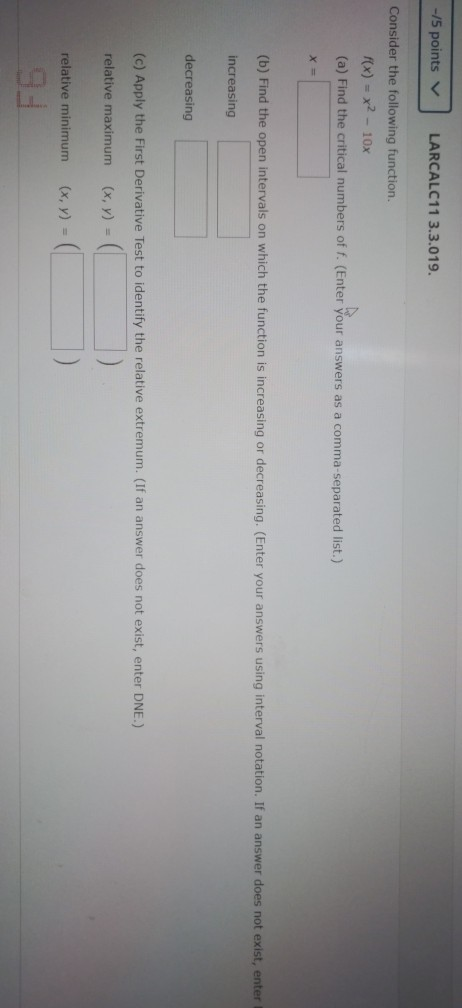-15 points LARCALC11 3.3.019. Consider the following function. f(x) = x2 - 10x (a) Find the critical numbers of f. (Enter your answers as a comma-separated list.) (b) Find the open intervals on which the function is increasing or decreasing. (Enter your answers using interval notation. If an answer does not exist, enter increasing decreasing (c) Apply the First Derivative Test to identify the relative extremum. (If an answer does not exist, enter DNE.) relative maximum (x, y) = relative...

• ### 14 points LarApCalc10 3.1.048 12. Consider the following. y--+4, +2x x0 Find the critical numbers. (Hint:...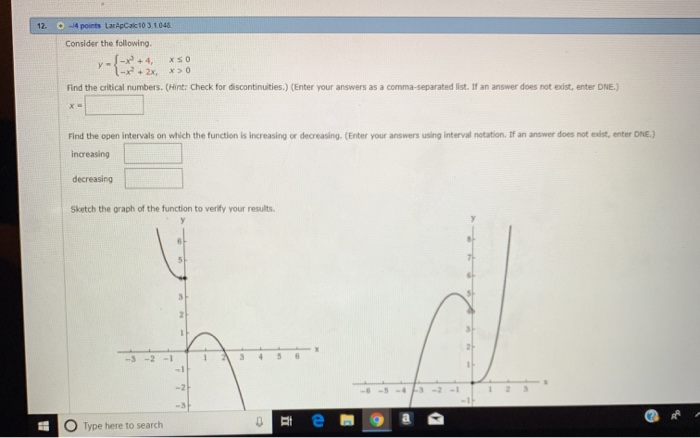14 points LarApCalc10 3.1.048 12. Consider the following. y--+4, +2x x0 Find the critical numbers. (Hint: Check for discontinuities.) (Enter your answers as a comma-separated list. If an answer does not exist, enter DNE.) Find the open intervals on which the function is increasing or decreasing. (Enter your answers using interval notation If an answer does not exist, enter DNE.) increasing decreasing Sketch the graph of the function to verify your results. 5 -3 -2 -1 -6-5-43-2-11 1 23 Type...

• ### Consider the following function. f(x) = cos(x) - sin(x), (0, 2) (a) Find the critical numbers...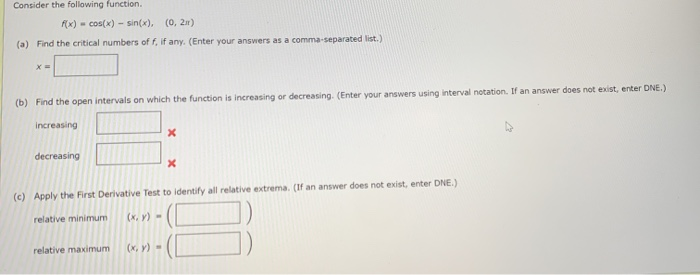Consider the following function. f(x) = cos(x) - sin(x), (0, 2) (a) Find the critical numbers of f, if any. (Enter your answers as a comma-separated list.) (b) Find the open intervals on which the function is increasing or decreasing (Enter your answers using interval notation. If an answer does not exist, enter DNE.) increasing х decreasing X (c) Apply the First Derivative Test to identify all relative extrema. (If an answer does not exist, enter DNE.) relative minimum (X,Y)...

• ### Consider the following function. f(x) = 2x3 + 3.r? – 120. (a) Find the critical numbers...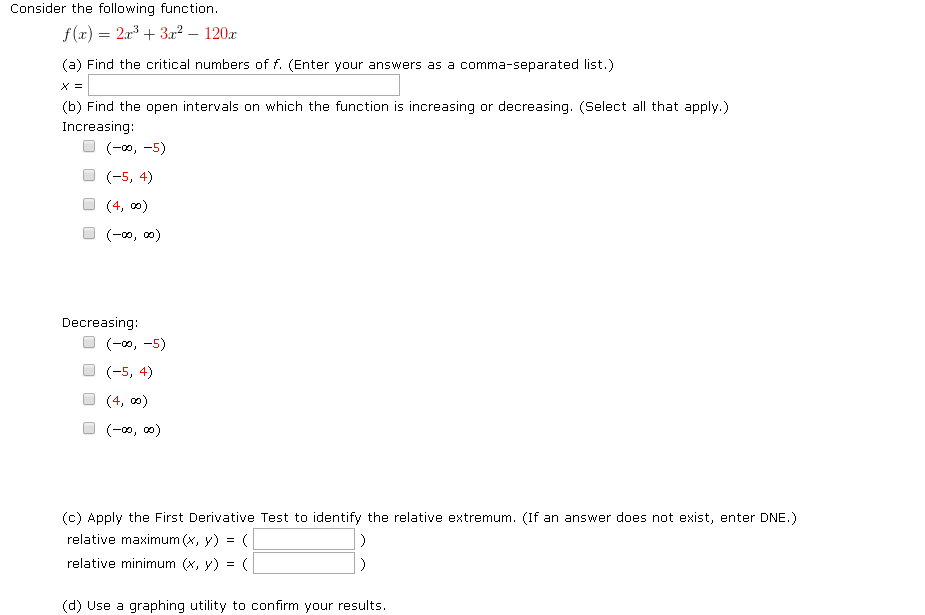Consider the following function. f(x) = 2x3 + 3.r? – 120. (a) Find the critical numbers of f. (Enter your answers as a comma-separated list.) (b) Find the open intervals on which the function is increasing or decreasing. (Select all that apply.) Increasing: (-9,-5) (-5, 4) (4,0) (-00,00) Decreasing: (-, -5) (-5, 4) (4,-) (-09, ) (C) Apply the First Derivative Test to identify the relative extremum. (If an answer does not exist, enter DNE.) relative maximum (x, y) =...

• ### Find the critical numbers of the function. (Enter your answers as a comma-separated list. If an...

Find the critical numbers of the function. (Enter your answers as a comma-separated list. If an answer does not exist, enter DNE. 1a. f(x) = 48x − 4x2 1b. g(x) = x4 − 2x2 + 2 1c.y = x x2 + 49 1d. f(x) = 9 − 9x, Find the open intervals on which the function is increasing or decreasing. Use a graphing utility to verify your results. (Enter your answers using interval notation. If an answer does not exist,...

• ### Find the critical points of the function and use the First Derivative Test to determine whether...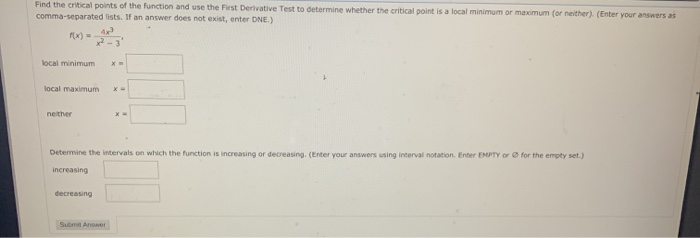Find the critical points of the function and use the First Derivative Test to determine whether the critical point is a local minimum or maximum (or neither). (Enter your answers as comma-separated lists. If an answer does not exist, enter DNE.) FX) 23 local minimum X local maximum X- neither Determine the intervals on which the function is increasing or decreasing, (Enter your answers using interval notation Enter EMPTY or for the empty set.) increasing decreasing Submit Answer

• ### Consider the following function (a) Find the critical numbers of any (Enter your answers as a...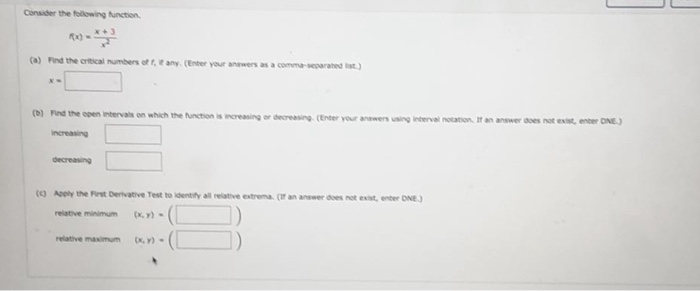Consider the following function (a) Find the critical numbers of any (Enter your answers as a comm o rated it) () Find the open intervals on which the function is increasing or decreasing (Enter your answers i nterval notation at an answer does not existente ONE) Increasing decreasing n ter ONE.) (0) Acolytheist Derivative Test to identify all relative extrema (tananswer does not e relative minimum Y)-(D relative maximum .Y)-(

• ### Find the critical points and the intervals on which the function f(t)=2-3«/, (x > 0) is...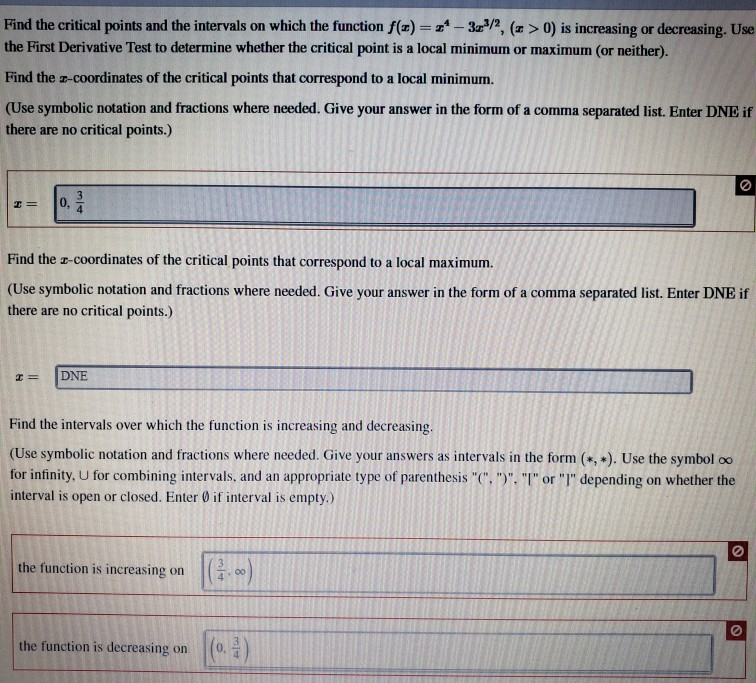Find the critical points and the intervals on which the function f(t)=2-3«/, (x > 0) is increasing or decreasing. Use the First Derivative Test to determine whether the critical point is a local minimum or maximum (or neither). Find the 2-coordinates of the critical points that correspond to a local minimum. (Use symbolic notation and fractions where needed. Give your answer in the form of a comma separated list. Enter DNE if there are no critical points.) Find the -coordinates...

• ### Find the critical numbers show all work Find the critical numbers of the function. (Enter your...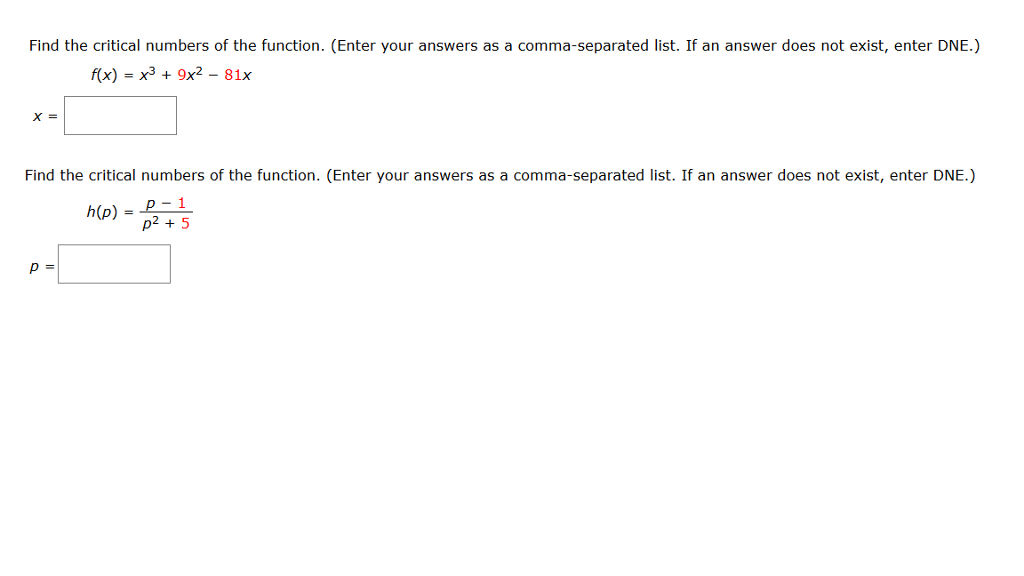Find the critical numbers show all work Find the critical numbers of the function. (Enter your answers as a comma-separated list. If an answer does not exist, enter DNE.) f(x) = x^3 + 9x^2 - 81x x = Find the critical numbers of the function. (Enter your answers as a comma-separated list. If an answer does not exist, enter DNE.) h(p) = p-1/p^2+5 p =